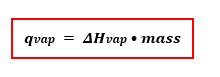Chemistry Practice Problems Heating and Cooling Curves Practice Problems Solution: A 75.0 mL sample of water is heated to its boiling...

🤓 Based on our data, we think this question is relevant for Professor Kennedy's class at GSU.

# Solution: A 75.0 mL sample of water is heated to its boiling point. How much heat is required to vaporize it? (Assume a density of 1.00 g/mL.)

###### Problem
A 75.0 mL sample of water is heated to its boiling point. How much heat is required to vaporize it? (Assume a density of 1.00 g/mL.)
###### Solution

Recall that the Heat required to vaporize or qvap can be solved using the enthalpy of vaporization of water.

The equation is:View Complete Written Solution

Heating and Cooling Curves

Heating and Cooling Curves

#### Q. Assume that the values given here for 1 mole of benzene are constant over the relevant temperature ranges.Melting point5.4 oCBoiling point90.1 oCΔHfus...

Solved • Tue Nov 27 2018 21:32:34 GMT-0500 (EST)

Heating and Cooling Curves

#### Q. Assume that the values given here for 1 mole of methanol are constant over the relevant temperature ranges.Melting Point176 KBoiling Point338 KHfus2.2...

Solved • Tue Nov 27 2018 21:32:34 GMT-0500 (EST)

Heating and Cooling Curves

#### Q. Assume that the values given here for 1 mole of methanol are constant over the relevant temperature ranges.Melting Point: 176 KBoiling Point: 338 KHfu...

Solved • Tue Nov 27 2018 21:32:34 GMT-0500 (EST)

Heating and Cooling Curves

#### Q. Calculate the amount of heat required to completely sublime 56.0 g of solid dry ice (CO2 ) at its sublimation temperature. The heat of sublimation for...

Solved • Tue Nov 27 2018 21:32:31 GMT-0500 (EST)